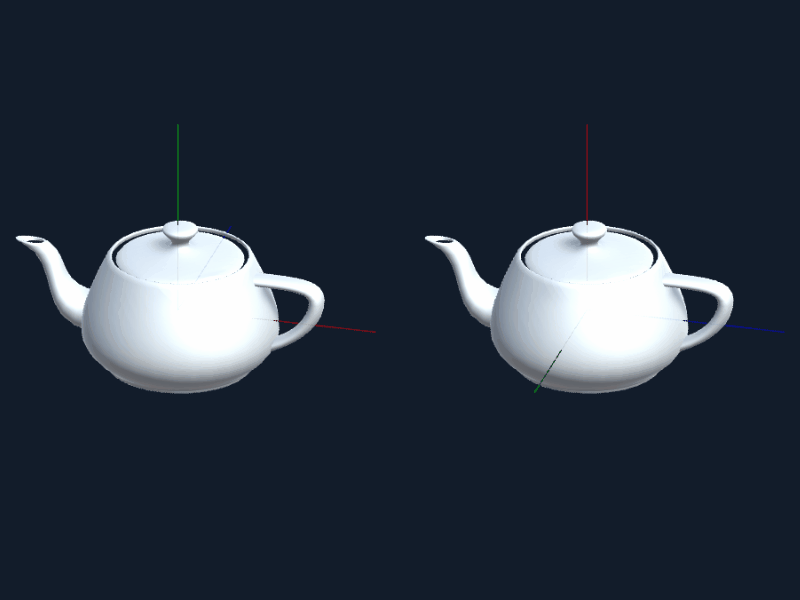Coordinate Frame Converter by gkjohnson - 4

A Unity utility for simply converting between different coordinate frames.

Unity 2017.1.1f1Apache License 2.0Updated 1 year agoCreated on November 14th, 2017
Go to source

# coordinate-frame-converter

A Unity utility for simply converting between different coordinate frames.Showing difference in euler angle application for frame `+X+Y-Z, -Z-X-Y` (left) and `+Z+X+Y, +X+Y+X` (right).

The angles `40, -220, -105` in the first frame are equivelant to `-40, -100, -150` in the second.

## Considerations

### Axis Direction

Which way is notionally considered “up”, “right”, and “forward”:

`````` U
|   F
| ／
.-----R
``````

### Rotation Order

Which order Euler angles are applied.

### Rotation Direction

Whether positive rotation is clockwise or counterclockwise about the “right hand rule”. Counterclockwise rotations are considered to be negative.

### Extrinsic and Intrinsic Rotations

Angles are applied using extrinsic or fixed axis rotations by default. To use intrinsic or moving axis rotations, simply invert the order in which rotations are applied.

## Use

``````using FrameConversions;

//...

// Unity forward is _into_ the screen, and uses left handed rotations
CoordinateFrame UnityFrame = new CoordinateFrame("+X+Y-Z", "-Z-X-Y");
CoordinateFrame OtherFrame = new CoordinateFrame("XYZ", "XYZ");

// Convert a position from the other coordinate frame into Unity's
OtherFrame.ConvertPosition(UnityFrame, new Vector3(1, 3, 2));

// Convert a set of euler angles from Unity Frame into the other frame
UnityFrame.ConvertEulerAngles(OtherFrame, new EulerAngles(20, 30, 40));

// Wrapper for converting between frames
CoordinateFrameConverter Unity2Other = new CoordinateFrameConverter(UnityFrame, OtherFrame);
Unity2Other.ConvertPosition(new Vector3(1,2,3));

// Converting from the other frame to Unity's
Unity2Other.inverse.ConvertPosition(new Vector3(1,2,3));
``````

## API

### CoordinateFrame

#### Constructor

``````CoordinateFrame( string rightUpForwardAxes, string rotationOrder )
``````

Specify the coordinate axes that define the vector used for `RIGHT`, `UP`, and `FORWARD` (out of the screen). The rotation order is specified with a similar string indicating the order in which rotations occur in the frame using fixed axes. The rotations are assumed to be right handed so if a left handed rotation is needed then negate the rotation:

``````// Left handed coordinate frame with left handed rotations.
new CoordinateFrame( "+X+Y-Z", "-Z-X-Y" );

// Right handed coordinate frame with right handed rotations.
new CoordinateFrame("XYZ", "XYZ")
``````

#### ConvertPosition

``````Vector3 ConvertPosition( CoordinateFrame targetFrame, Vector3 position )
``````

Converts the given position into the target frame.

#### ConvertEulerAngles

``````Euler ConvertEulerAngles( CoordinateFrame targetFrame, Euler angles )
``````

Converts the given angles into the given frames rotation order.

## Structs

### Unity.Vector3

Represents the position in a coordinate frame. Specifys a point `x` units on the X axis, `y` units on the Y axis, and `z` units on the Z axis in the particular frame the point is in.

### EulerAngles

Represents the ordered rotation in a coordinate frame. Specifies the order in degrees a rotation should occur about the first, second, then third rotation axis as specified in a rotation order axis set.

## Gotchas

• Unity takes euler orders as a vector and applies them in Z, X, Y order (taking them from place 2, then 0, then 1 from the vector). This library assumes the angles in EulerAngles are specified in the order they are applied, so Unity’s would have to be specified as [Z, X, Y] in the struct. So Euler Angles specified as `Vector3(10, 20, 30)` in Unity would have to be specified as `EulerAngles(30, 10, 20)`
Show all projects by gkjohnson